Open in App
Not now

# Reading Tabular Data from files in R Programming

• Last Updated : 10 May, 2020

Often, the data which is to be read and worked upon is already stored in a file but is present outside the R environment. Hence, importing data into R is a mandatory task in such circumstances. The formats which are supported by R are CSV, JSON, Excel, Text, XML, etc. The majority of times, the data to be read into R is in tabular format. The functions used for reading such data, which is stored in the form of rows and columns, import the data and return data frame in R. Data frame is preferred in R because it is easier to extract data from rows and columns of a data frame for statistical computation tasks than other data structures in R. The most common functions which are used for reading tabular data into R are:- read.table(), read.csv(), fromJSON() and read.xlxs() .

#### Reading Data from Text File

Functions used for reading tabular data from a text file is `read.table()`

Parameters:

• file: Specifies the name of the file.
• header:The header is a logical flag indicating whether the first line is a header line contains data or not.
• nrows: Specifies number of rows in the dataset.
• skip: Helps in skipping of lines from the beginning.
• colClasses: It is a character vector which indicates class of each column of the data set.
• sep: It a string indicating the way the columns are separated that is by commas, spaces, colons, tabs etc.

For small or moderately sized data sets, we can call read.table() without any arguments. R will automatically figure out the number of rows, the number of columns, classes of different columns, skip lines that start with #(comment symbol), etc. If we do specify the arguments, it will make the execution faster and efficient but here, since the dataset is small so it would not make much of a difference as it is already fast and efficient.

Example:
Let there be a tabular data file GeeksforGeeks.txt saved in the current directory with data as follows: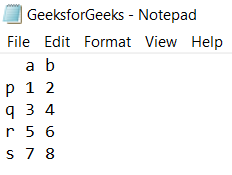`read.table(``"GeeksforGeeks.txt"``)`

Output: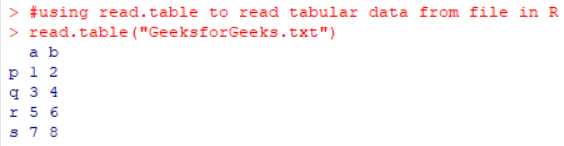#### Reading Data from a CSV File

`read.csv()` function is used to read .csv files which is a very common format followed by spreadsheet applications like Microsoft Excel. read.csv() is similar to read.table() except that the default separator for the read.csv() function is the comma, whereas the default separator for read.table() is the space. The other thing that read.csv() specifies is that it always specified header to be equal to true.

While reading huge files, a point to keep in mind is to calculate the approximate memory required for it to store the dataset. It is to ensure that it is not more than the RAM available in the device we are working in. The memory required can be calculated as shown below:

```Consider the table has 2000000 rows and 200 columns considering if all the columns are of class numeric.

2000000 x 200 x 8 bytes/numeric #each number requires 8 bytes to be stored
=3200000000/bytes/MB
=3051.76/MB
=2.98 GB

Approximately twice this amount of RAM i.e.5.96 GB will be required.
```

Another measure that can be followed to reduce time consumption is including the colClasses argument so that R does not have to search the class of each column of the dataset. If the number of rows i.e. nrows are specified beforehand then it will be helpful in reducing memory usage.

Example:
Let there be a tabular data file GeeksforGeeks.csv saved in the current directory with data as follows: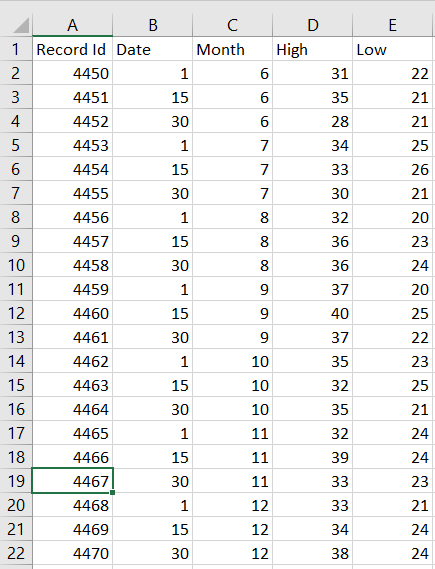`read.csv(``"GeeksforGeeks.csv"``)`

Output :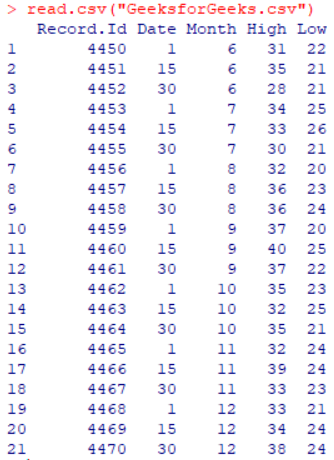#### Reading data from JSON File

`fromJSON()` function is used to convert JSON data to R objects. This function requires installation of rjson package. This can be done by the following command:

`install.packages("rjson")`

Example:
Let there be a .json file GeeksforGeeks.json saved in the current directory with content as follows: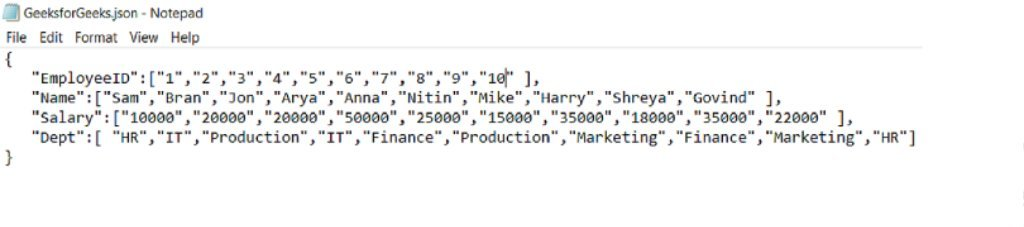`library(rjson) ``#loads the rjson library``fromJSON(``file``=``"GeeksforGeeks.json"``)`

Output: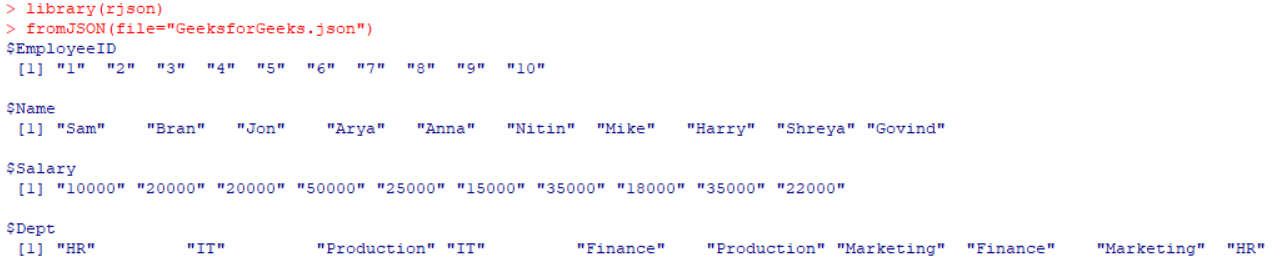`as.data.frame(fromJSON(``file``=``"GeeksforGeeks.json"``))`

Output: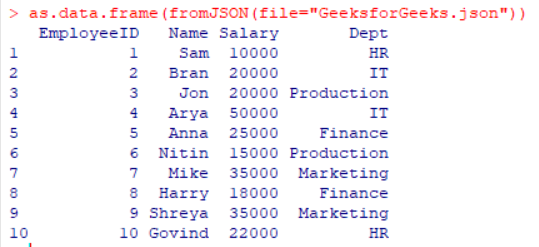The R function `read.xlsx()` is used to read the contents of an Excel worksheet into a R data frame. This function requires installation of xlsx package. This can be done by using the following command:

`install.packages("xlsx")`

Example:
Let there be an Excel file gfg.xlsx saved in the current directory with content as follows:`library(``"xlsx"``) ``#loads the xlsx library``read.xlsx(``"gfg.xlsx"``, ``1``) ``#here 1 represents the sheet number`

Output: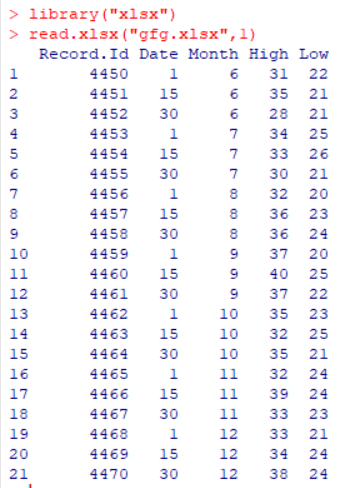Here, we can observe that the `read.xlsx()` has returned a data.frame as output.

But if large data sets (with more than 100000 cells) are to be read, then using read.xlsx2() is preferred. read.xlsx2() works faster on large files than read.xlsx().The result of read.xlsx2() will be different from read.xlsx(), because internally read.xlsx2() uses readColumns() which is tailored for tabular data.

My Personal Notes arrow_drop_up